# Test: Standard Deviation

## 10 Questions MCQ Test Quantitative Aptitude for GMAT | Test: Standard Deviation

Description
Attempt Test: Standard Deviation | 10 questions in 10 minutes | Mock test for GMAT preparation | Free important questions MCQ to study Quantitative Aptitude for GMAT for GMAT Exam | Download free PDF with solutions
QUESTION: 1

Solution:
QUESTION: 2

Solution:
QUESTION: 3

### If M is a negative integer and K is a positive integer, which of the following could be the standard deviation of a set {-7, -5, -3, M, 0, 1, 3, K, 7}? I. -1.5 II. -2 III. 0

Solution:
QUESTION: 4

Sets A, B and C are shown below. If number 100 is included in each of these sets, which of the following represents the correct ordering of the sets in terms of the absolute increase in their standard deviation, from largest to smallest?
A {30, 50, 70, 90, 110},
B {-20, -10, 0, 10, 20},
C {30, 35, 40, 45, 50}

Solution:
QUESTION: 5

The table below represents three sets of numbers with their respective medians, means and standard
deviations. The third set, Set [A+B], denotes the set that is formed by combining Set A and Set B.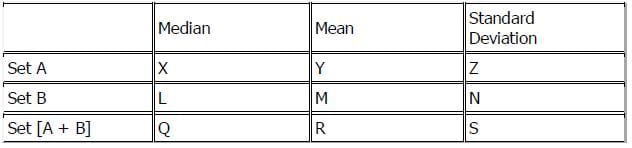If X – Y > 0 and L – M = 0, then which of the following must be true?
I. Z > N II. R > M III. Q > R

Solution:

If X – Y > 0, then X > Y and the median of A is greater than the mean of set A. If L – M = 0, then L = M and the median of set B is equal to the mean of set B.

I. NOT NECESSARILY: According to the table, Z > N means that the standard deviation of set A is greater than that of set B. Standard deviation is a measure of how close the terms of a given set are to the mean of the set. If a set has a high standard deviation, its terms are relatively far from the mean. If a set has a low standard deviation, its terms are relatively close to the mean.

Recall that a median separates the set into two as far as the number of terms. There is an equal number of terms both above and below the median. If the median of a set is greater than the mean, however, the terms below the median must collectively be farther from the median than the terms above the median.   For example, in the set {1, 89, 90}, the median is 89 and the mean is 60. The median is much greater than the mean because 1 is much farther from 89 than 90 is.

Knowing that the median of set A is greater than the mean of set A just tells us that the terms below set A’s median are further from the median than the terms above set A’s median. This does not necessarily imply that the terms, overall, are further away from the mean than in set B, where the terms below the median are the same distance from the median as the terms above it.   In fact, a set in which the mean and median are equal can have a very high standard deviation if the terms are both far below the mean and far above it.

II. NOT NECESSARILY: According to the table, R > M implies that the mean of set [A + B] is greater than the mean of set B. This is not necessarily true. When two sets are combined to form a composite set, the mean of the composite set must either be between the means of the individual sets or be equal to the mean of both of the individual sets.  To prove this, consider the simple example of one member sets: A = , B = , A + B = [3, 5]. In this case the mean of A + B is greater than the mean of A and less than the mean of B. We could easily have reversed this result by reversing the members of sets A and B.

III. NOT NECESSARILY: According to the table, Q > R implies that the median of the set [A + B] is greater than the mean of set [A + B].  We can extend the rule given in statement II to medians as well: when two sets are combined to form a composite set, the median of the composite set must either be between the medians of the individual sets or be equal to the median of one or both of the individual sets. While the median of set A is greater than the mean of set A and the median of set B is equal to the mean of set B, set [A + B] might have a median that is greater or less than the mean of set [A + B].   See the two tables for illustration: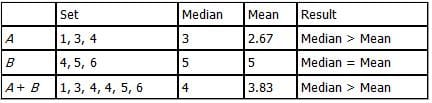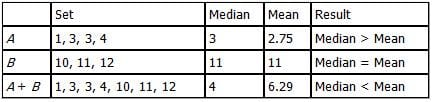Therefore none of the statements are necessarily true and the correct answer is E.

QUESTION: 6

Three fair coins are labeled with a zero (0) on one side and a one (1) on the other side. Jimmy flips all three coins at once and computes the sum of the numbers displayed. He does this over 1000 times, writing downthe sums in a long list. What is the expected standard deviation of the sums on this list?

Solution:

First, set up each coin in a column and compute the sum of each possible trial as follows: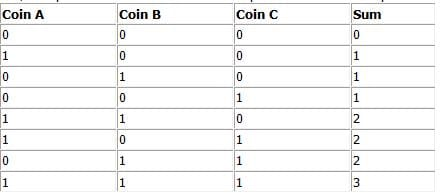Now compute the average (mean) of the sums using one of the following methods:
Method 1: Use the Average Rule (Average = Sum / Number of numbers).
(0 + 1 + 1 + 1 + 2 + 2 + 2 + 3) ÷ 8 = 12 ÷ 8 = 3/2
Method 2: Multiply each possible sum by its probability and add.
(0 × 1/8) + (1 × 3/8) + (2 × 3/8) + (3 × 1/8) = 12/8 = 3/2
Method 3: Since the sums have a symmetrical form, spot immediately that the mean must be right in the middle. You have one 0, three 1’s, three 2’s and one 3 – so the mean must be exactly in the middle = 1.5 or 3/2.

Then, to get the standard deviation, do the following:
(a) Compute the difference of each trial from the average of 3/2 that was just determined. (Technically it’s “average minus trial” but the sign does not matter since the result will be squared in the next step.)
(b) Square each of those differences.
(c) Find the average (mean) of those squared differences.
(d) Take the square root of this average.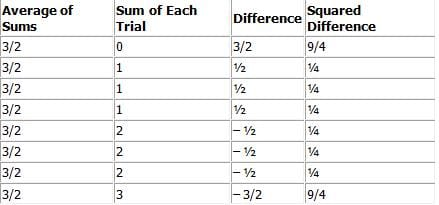The average of the squared differences = (9/4 + ¼ + ¼ + ¼ + ¼ + ¼ + ¼ + 9/4) ÷ 8 = 6 ÷ 8 = ¾.
Finally, the square root of this average = √3/2

Note: When you compute averages, be careful to count all trials (or equivalently, to take probabilities into account). For instance, if you simply take each unique difference that you find (3/2, ½, –1/2 and –3/2), square those and average them, you will get 5/4, and the standard deviation as √5/2
This is incorrect because it implies that the 3/2 and –3/2 differences are as common as the ½ and –1/2 differences. This is not true since the ½ and –1/2 differences occur three times as frequently as the 3/2 and –3/2 differences.

QUESTION: 7

Let Set T = {2, 4, 5, 7}. Which of the following values, if added to Set T, would most increase the standard deviation of Set T?

Solution:

Standard deviation is a measure of how far the data points in a set fall from the mean.  For example, {5, 5, 6, 7, 7} has a small standard deviation relative to {1, 4, 6, 7, 10}.  The values in the second set are much further from the mean than the values in the first set. In general, a value that drastically increases the range of a set will also have a large impact on the standard deviation.  In this case, 14 creates the largest spread of the five answer choices, and will therefore be the value that most increases the standard deviation of Set T. The correct answer is E.

QUESTION: 8

9.4, 9.9, 9.9, 9.9, 10.0, 10.2, 10.2, 10.5
The mean and the standard deviation of the 8 numbers shown are 10 and 0.3, respectively. What percentageof the 8 number's are within 1 standard deviation?

Solution:

10-0.3=9.7 10+0.3=10.3 the number within 1 standard deviation should be between 9.7-10.3 so there are six numbers within 1 standard deviation 6/8=75%

QUESTION: 9

70, 75,80,85,90,105,105,130,130,130
The list shown consists of the times, in seconds, that i took each of 10 schoolchildren to run a distance of 400 on of meters. If the standard devastation of the 10 running times is 22.4 seconds, rounded to the nearest tenth of a second, how many of the 10 running times are more than 1 standard deviation below the mean of the 10 running times?

Solution:

Average=100

1 standard deviation below the mean: less than 100-22.4=77.6

Obviously, 70 and 75 can fulfill the requirements.Use Code STAYHOME200 and get INR 200 additional OFF Use Coupon Code